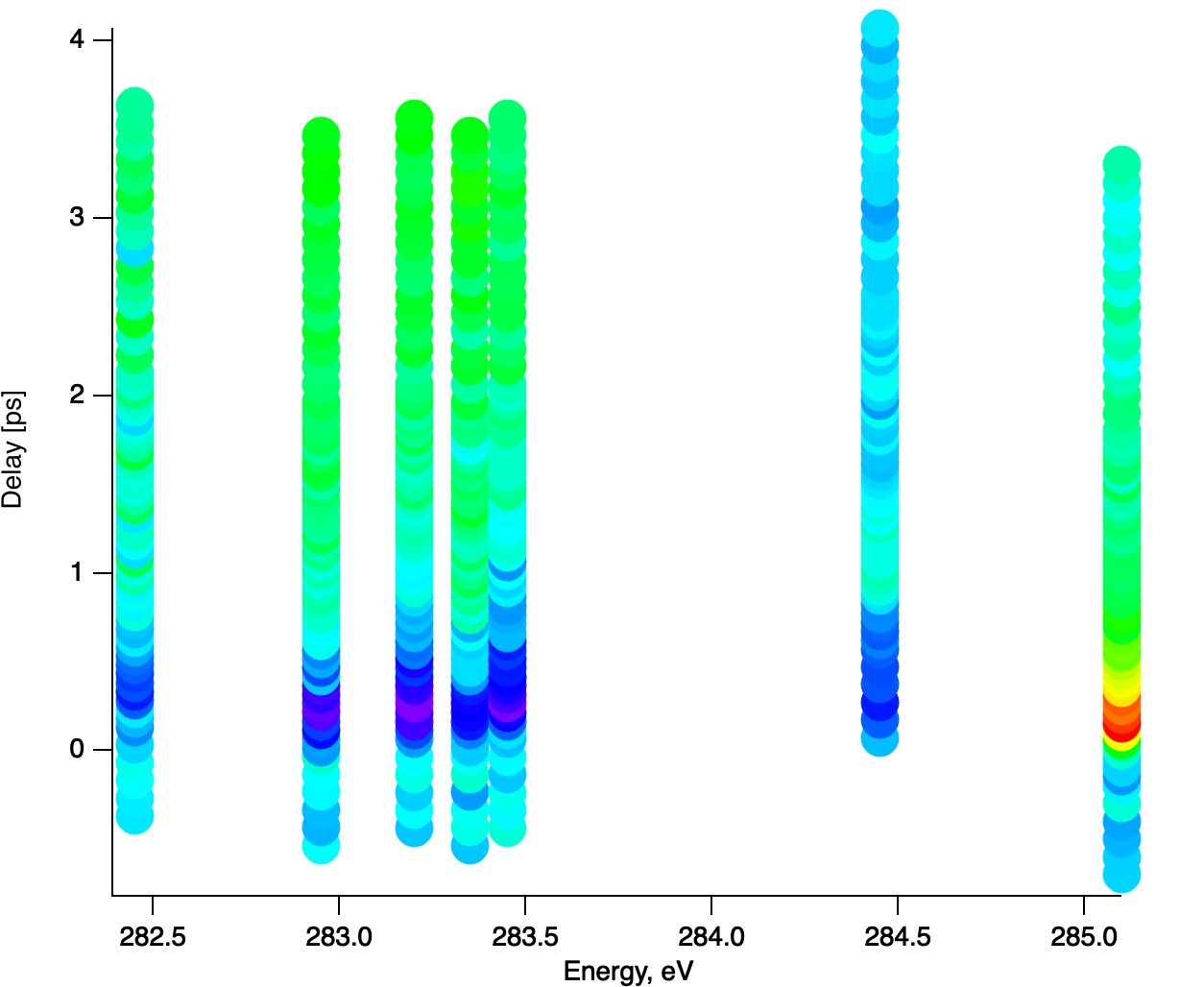# 2D plottingHi,

I have a little problem, I have bunch of waves that I want to do a 2D image of. The problem is that each y-wave has its own x-wave, that differs between each other, not much but differ. So how do I do a 2D where each 1D has the correct x value.

Thank you very much in advance for any contribution

Jörgen

Let's clarify a bit...

Are you saying you want to treat each XY pair as a raster line in an image, but you want the "pixels" in the image to be at the correct X position? So is this a false-color image? That is, intensity of each pixel is represented by a gray shade or a color? And is the intensity the Y value from your XY pair of waves? The whole concept of an image is pretty much based on a rectangular grid of pixels, so it's really not clear what you really want.

If I have understood, you could make a series of graph traces, using some constant Y (that's vertical position Y, not your Y) plotted vs your X wave. Use Markers mode, and choose a marker that gives a pleasing result, probably filled square or filled circle. Finally, use color as f(z) using your Y wave to set the color.

If you can answer the questions, I might even be able to make an example... Better yet, answer the questions and post an Igor experiment file with your data.

If the idea is, as it sounds, to take a bunch of 1D XY-data and merge it into a 2D wave then you can write a small script which looks up the x-points for each y-wave and transfers the data at the right position of a prepared 2D matrix. Note that this approach will interpolate the data a bit when the x-scaling is not perfectly aligned to the grid of the 2D matrix. Is the x data linear, so that you could in principle create waveform data (just one 1D with x-scaling applied) from it? Or you could first use the Interpolate function to transform your data from XY to waveform, if the scaling is not linear and the interpolation does not damage your data (the data will be forced into a linear grid anyway when merged into a 2D wave). It would get considerably easier when you just have to apply the correct x-shift before writing into the 2D wave. In fact, I already have a script which merges waveform data into a 2D matrix with the correct scaling. We can help you to better solve the problem or even prepare a script if you are willing to post some example data.

In reply to by johnweeks

Hi,

yes this is an false-color-plot. If you look at the graph that I have attached each of this waves has its own x wave "the delay", so yes XY pairs of waves, and what I want to do is to do a 2D plot or "false-color-plot" where I have the energies as the x-axis, the delays as the y-axis, and the intensity as the z-axis. I hope this is the answer of your questions.

Best

Jörgen

Well, here is what my scheme looks like. I'm not sure it's what you're after, though. Edited my post when I realized I got the ordering wrong.